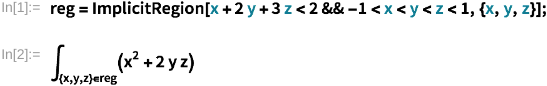Ask Your Question

# Triple integrals in a specific region of space

Can I perform a triple integral in a region of space I define? I'm trying to migrate from Mathematica to Sage, and in Mathematica I could go and define a region of space (with various limitations) and then perform the integral of a function on it.

In Mathematica:

reg = ImplicitRegion[x + 2 y + 3 z < 2 && -1 < x < y < z < 1, {x, y, z}];
integral[{x, y, z} in reg, (x^2 + 2 y z)]Is there a way to easily perform this operation even in Sage?

edit retag close merge delete

1

## 1 Answer

Sort by » oldest newest most voted

## Integrate a polynomial over a polyhedron

### Overview

The question is about integrating a polynomial over a region defined by linear inequalities.

Such a region is called a polytope or a polyhedron.

In Sage one can construct such regions using the Polyhedron class.

This class provides a method integrate which allows, when the latte_int optional package is installed, to integrate a polynomial function over a polyhedron.

In a way, the trick is: rather than start from the function integrate and somehow specify a region, we fist define a polyhedron and then use the method integrate to compute the integral of a polynomial over that polyhedron.

### Steps

The region is defined by five inequalities:

x + 2 y + 3 z < 2
-1 < x
x < y
y < z
z < 1


and the function to integrate is

x^2 + 2 y z


The five inequalities can be rewritten as

2 + (-1) * x + (-2) * y + (-3) * z > 0
1 + (+1) * x                       > 0
0 + (-1) * x + (+1) * y            > 0
0            + (-1) * y + (+1) * z > 0
1                       + (-1) * z > 0


Encode these inequalities in Sage as

sage: ieqs = [[2, -1, -2, -3],
....:         [1,  1,  0,  0],
....:         [0, -1,  1,  0],
....:         [0,  0, -1,  1],
....:         [1,  0,  0, -1], ]


Create a polyhedron from those inequalities:

sage: P = Polyhedron(ieqs=ieqs)


Define polynomial variables and a polynomial:

sage: x, y, z = polygens(QQ, names='x, y, z')
sage: f = x^2 + 2*y*z


Integrate that polynomial over the polyhedron we defined:

sage: P.integrate(f)
53833/151875


This last command requires the latte_int optional package for Sage to be installed.

### The latte_int optional package

To install that package, if you installed Sage from source or from binaries downloaded from the Sage website, run the following command in a terminal:

\$ sage -i latte_int


more

## Your Answer

Please start posting anonymously - your entry will be published after you log in or create a new account.

Add Answer

## Stats

Asked: 2020-09-12 12:50:48 +0200

Seen: 184 times

Last updated: Oct 11 '20